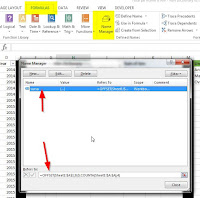## joi, 4 iunie 2015

### 3 methods to summarize total amounts per users and years in Excel 2013 [Pivot Table, Dictionaries, Arrays]Suppose that we have some raw data like in the image and we need to summarize it in Excel by Names and Years. Although in most of the cases the easiest way to complete this task is by using an Excel Pivot Table or SUMIF/SUMIFS formulas, there might be cases when we will want/need to use other methods. (especially when creating VBA scripts for process automation).

In this post i will present 2 fast alternatives which i used lately and a comparison regarding the speed. Which method will be the fastest: the Pivot Table, the VBA Dictionaries or the VBA Variant Arrays ? You will  also find the sample file download link at the end of the post.

Method 1: VBA Dictionaries. You can read more about them in this article. The code i used to summarize more than 135.000 rows of data using this method is the following:
```Sub test_d()

Dim namesdictionary As Dictionary
Dim yearsdictionary As Dictionary

Set namesdictionary = New Dictionary '-main dictionary with names
Set yearsdictionary = New Dictionary '-secondary dictionary with years
Set ws = ActiveSheet
lr = ws.Cells(ws.Rows.Count, "A").End(xlUp).Row

StartTime = Timer

ws.Range("F3:H1000").ClearContents

For i = 2 To lr

dKey = ws.Range("A" & i)
damount = ws.Range("B" & i)
dyear = ws.Range("D" & i)

If namesdictionary.Exists(dKey) Then 'if the name already exists then set yearsdictionary in namesdictionary with the key from the row

Set yearsdictionary = namesdictionary(dKey)
If yearsdictionary.Exists(dyear) Then         'check if the year already exists in yearsdictionary and if is true then add the amount from the row to the yearsdictionary amount

damount = damount + yearsdictionary(dyear)
yearsdictionary(dyear) = damount

Else
yearsdictionary.Add dyear, damount     'if it doesn't exist then add new years dictionary with the row amount

End If

Else                                'if the name does not exist then add yearsdictionary and namesdictionary for that row

Set yearsdictionary = New Dictionary

End If

Next i

'scrie valorile
r = 3
For Each dKey In namesdictionary.Keys

Set yearsdictionary = namesdictionary(dKey)

For Each dyear In yearsdictionary.Keys

ws.Range("F" & r) = dKey
ws.Range("G" & r) = dyear
ws.Range("H" & r) = yearsdictionary(dyear)
r = r + 1

Next dyear

Next dKey

'set dictionaries to nothing to release the memory
Set namesdictionary = Nothing
Set yearsdictionary = Nothing

SecondsElapsed = Round(Timer - StartTime, 2)
ws.Range("F15") = "Time: " & SecondsElapsed & " seconds"
ws.Range("F15").Font.Italic = True
ws.Range("F15:H15").Interior.Color = RGB(255, 128, 128)
ws.Range("F16") = "No of rows: " & lr

End Sub
```

Method 2: VBA Variant Arrays. More about them in this article. The code for the same data:
```Sub test_array()

Set ws = ActiveSheet
lr = ws.Cells(ws.Rows.Count, "A").End(xlUp).Row
Dim x() As Variant, y() As Variant

StartTime = Timer

ws.Range("R3:T1000").ClearContents      'clear old data

'copy data
ws.Range("A2:A" & lr).Copy ws.Range("R3")
ws.Range("D2:D" & lr).Copy ws.Range("S3")
Application.CutCopyMode = False

'remove duplicates
ws.Range("\$R\$2:\$S\$" & lr + 1).RemoveDuplicates Columns:=Array(1, 2), Header _
:=xlYes

x = ws.Range("A2:D" & lr).Value                                         'array with gross data
y = ws.Range("R3:T" & ws.Cells(ws.Rows.Count, "R").End(xlUp).Row).Value      'array with summarized data

'loop through arrays and summarize amounts by names and years
amount = 0
For i = LBound(y) To UBound(y)
For j = LBound(x) To UBound(x)

If y(i, 1) = x(j, 1) And y(i, 2) = x(j, 4) Then
amount = amount + x(j, 2)
End If

Next j

y(i, 3) = amount
amount = 0

Next i

'write array to sheet including the summarized amounts
ws.Range("R3:T" & ws.Cells(ws.Rows.Count, "R").End(xlUp).Row) = y

Erase x()
Erase y()

SecondsElapsed = Round(Timer - StartTime, 2)
ws.Range("R15") = "Time: " & SecondsElapsed & " seconds"
ws.Range("R15").Font.Italic = True
ws.Range("R15:T15").Interior.Color = RGB(255, 128, 128)

End Sub
```Method 3: Excel Pivot Table with dynamic source data range. The formula for the source data range is the following:" =OFFSET(Sheet1!\$A\$1;0;0;COUNTA(Sheet1!\$A:\$A);4)" . This named range is defined in Excel Formulas --> Name manager and then is set as source data range for the pivot table. After this, when you add more rows in the raw data range and refresh the pivot table, the source will be updated dynamicaly.The speed results for more than 135.000 rows of sample data, core i5 laptop with 16 gb RAM (the variances are significant depending on the computer performance):1) Pivot Table (only refresh): 0.19 seconds.
2) VBA Variant Arrays: 0.81 seconds.
3) VBA Dictionaries: 2.78 seconds. Although this was the slowest method, in other situations it can be very useful and it is also very interesting.

Code for using dictionaries to take only unique values:
```Sub unique_names()

Dim namesdictionary As Dictionary
Set namesdictionary = New Dictionary
Set ws = ActiveSheet
lr = ws.Cells(ws.Rows.Count, "A").End(xlUp).Row

ws.Range("N2:N100").ClearContents

'insert unique values to dictionary
For i = 2 To lr

On Error Resume Next
namesdictionary.Add ws.Range("A" & i).Value, 1
On Error GoTo 0

Next i

'write values to column N
r = 2
For Each dKey In namesdictionary.Keys
ws.Range("N" & r) = dKey
r = r + 1
Next

End Sub
```

Code to refresh the pivot table in VBA:
```Sub refresh_pt()

Set ws = ActiveSheet

StartTime = Timer

'refresh pivot
For Each pt In ws.PivotTables

pt.RefreshTable

Next pt

SecondsElapsed = Round(Timer - StartTime, 2)
ws.Range("J15") = "Time: " & SecondsElapsed & " seconds"
ws.Range("J15").Font.Italic = True
ws.Range("J15:L15").Interior.Color = RGB(255, 128, 128)

End Sub
```

1.